Get inspired by the success stories of our students in IIT JAM MS, ISI  MStat, CMI MSc DS.  Learn More

# Size, Power, and Condition | ISI MStat 2019 PSB Problem 9This is a problem from the ISI MStat Entrance Examination, 2019. This primarily tests one's familiarity with size, power of a test and whether he/she is able to condition an event properly.

## The Problem:

Let Z be a random variable with probability density function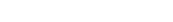with parameter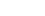. Suppose, we observe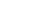max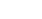.

(a)Find the constant c such that the test that "rejects when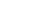" has size 0.05 for the null hypothesis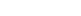.

(b)Find the power of this test against the alternative hypothesis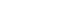.

## Prerequisites:

• A thorough knowledge about the size and power of a test
• Having a good sense of conditioning whenever a function (like max()) is defined piecewise.

And believe me as Joe Blitzstein says: "Conditioning is the soul of statistics"

## Solution:

(a) If you know what size of a test means, then you can easily write down the condition mentioned in part(a) in mathematical terms.

It simply means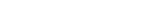Now, under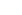,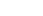.

So, we have the pdf of Z as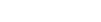As the support of Z is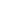, we can partition it in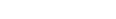.

Now, let's condition based on this partition. So, we have: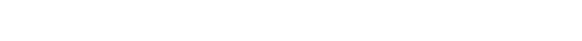Do, you understand the last equality? (Try to convince yourself why)

So,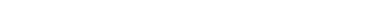Equating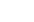with 0.05, we get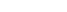(b) The second part is just mere calculation given already you know the value of c.

Power of test against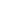is given by: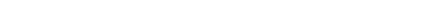## Try out this one:

The pdf occurring in this problem is an example of a Laplace distribution.Look it up on the internet if you are not aware and go through its properties.

Suppose you have a random variable V which follows Exponential Distribution with mean 1.

Let I be a Bernoulli() random variable. It is given that I,V are independent.

Can you find a function h (which is also a random variable),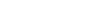( a continuous function of I and V) such that h has the standard Laplace distribution?

This is a problem from the ISI MStat Entrance Examination, 2019. This primarily tests one's familiarity with size, power of a test and whether he/she is able to condition an event properly.

## The Problem:

Let Z be a random variable with probability density functionwith parameter. Suppose, we observemax.

(a)Find the constant c such that the test that "rejects when" has size 0.05 for the null hypothesis.

(b)Find the power of this test against the alternative hypothesis.

## Prerequisites:

• A thorough knowledge about the size and power of a test
• Having a good sense of conditioning whenever a function (like max()) is defined piecewise.

And believe me as Joe Blitzstein says: "Conditioning is the soul of statistics"

## Solution:

(a) If you know what size of a test means, then you can easily write down the condition mentioned in part(a) in mathematical terms.

It simply meansNow, under,.

So, we have the pdf of Z asAs the support of Z is, we can partition it in.

Now, let's condition based on this partition. So, we have:Do, you understand the last equality? (Try to convince yourself why)

So,Equatingwith 0.05, we get(b) The second part is just mere calculation given already you know the value of c.

Power of test againstis given by:## Try out this one:

The pdf occurring in this problem is an example of a Laplace distribution.Look it up on the internet if you are not aware and go through its properties.

Suppose you have a random variable V which follows Exponential Distribution with mean 1.

Let I be a Bernoulli() random variable. It is given that I,V are independent.

Can you find a function h (which is also a random variable),( a continuous function of I and V) such that h has the standard Laplace distribution?

This site uses Akismet to reduce spam. Learn how your comment data is processed.

### 2 comments on “Size, Power, and Condition | ISI MStat 2019 PSB Problem 9”

1.Arnab Chattopadhyay. says:

Take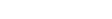Now since I can take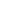orand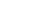so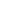can take any real numbers as it's value. Let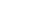Then by using independence of the random variablesand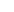we getNow if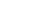then again by using independence of the random variablesandwe getThus the random variablefollows standard laplace distribution.

1.Srijit Mukherjee says:

Great Work Arnab. Stay Tuned!

### Knowledge Partner